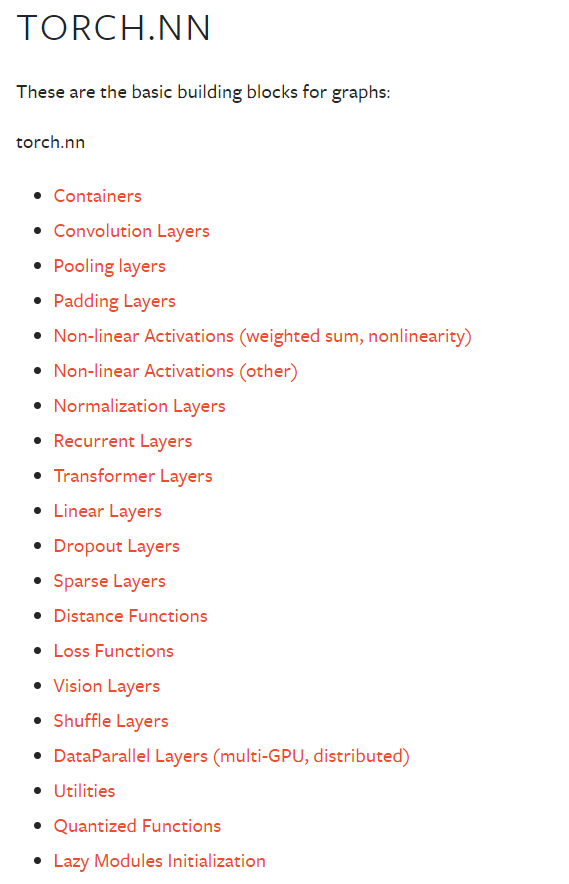Pytorch入门中 —— 搭建网络模型

2021-12-16卷积层in_channels (int)：输入数据的通道数，图片通常为3
out_channels (int)：输出数据的通道数，也是卷积核的个数
kernel_size (int or tuple)：卷积核大小，传入int表示正方形，传入tuple代表高和宽
stride (int or tuple, optional)：卷积操作的步长，传入int代表横向和纵向步长相同，默认为1
padding (int, tuple or str, optional)：填充厚度，传入int代表上下左右四个边填充厚度相同，默认为0，即不填充$H_{out} = \left\lfloor\frac{H_{in} + 2 \times \text{padding} -\text{kernel\_size}}{\text{stride}} + 1\right\rfloor$$W_{out} = \left\lfloor\frac{W_{in} + 2 \times \text{padding} - \text{kernel\_size}}{\text{stride}} + 1\right\rfloor$池化层kernel_size：池化操作时的窗口大小
stride：池化操作时的步长，默认为kernel_size

非线性激活线性层in_features：输入特征大小
out_features：输出特征大小
bias：是否添加偏置，默认为True

模型搭建示例1.由于CIFAR10数据集中图片为3*32*32，所以图中模型的输入为3通道，高宽都为32的张量。

2.使用 5*5的卷积核进行卷积操作，得到通道数为32，高和宽为32的张量。因此我们可以推出该卷积层的参数如下：

in_channels = 3
out_channels = 32
kernel_size = 5
stride = 1$H_{out} = \left\lfloor\frac{H_{in} + 2 \times \text{padding} -\text{kernel\_size}}{\text{stride}} + 1\right\rfloor$$32 = \left\lfloor\frac{32 + 2 \times \text{padding} -\text{5}}{\text{stride}} + 1\right\rfloor$3.使用2*2的核进行最大池化操作，得到通道数为32，高和宽为16的张量。可以推出该池化层的参数如下：

kernel_size = 2
stride = 2

4.使用 5*5的卷积核进行卷积操作，得到通道数为32，高和宽为16的张量。因此我们可以推出该卷积层的参数如下：

in_channels = 32
out_channels = 32
kernel_size = 5
stride = 1

5.使用2*2的核进行最大池化操作，得到通道数为32，高和宽为8的张量。可以推出该池化层的参数如下：

kernel_size = 2
stride = 2

6.使用 5*5的卷积核进行卷积操作，得到通道数为64，高和宽为8的张量。因此我们可以推出该卷积层的参数如下：

in_channels = 32
out_channels = 64
kernel_size = 5
stride = 1

7.使用2*2的核进行最大池化操作，得到通道数为64，高和宽为4的张量。可以推出该池化层的参数如下：

kernel_size = 2
stride = 2

8.将64*4*4的张量进行展平操作得到长为1024的向量。

9.将长为1024的向量进行线性变换得到长为64的向量（隐藏层），可以推出该线性层的参数如下：

in_features：1024
out_features：64

10.将长为64的向量进行线性变换得到长为10的向量，可以推出该线性层的参数如下：

in_features：64
out_features：10

from torch import nn

class MyModel(nn.Module):
def __init__(self):
super(MyModel, self).__init__()
self.conv1 = nn.Conv2d(3, 32, 5, padding=2)
self.max_pool1 = nn.MaxPool2d(2)
self.conv2 = nn.Conv2d(32, 32, 5, padding=2)
self.max_pool2 = nn.MaxPool2d(2)
self.conv3 = nn.Conv2d(32, 64, 5, padding=2)
self.max_pool3 = nn.MaxPool2d(2)
self.flatten = nn.Flatten()
self.linear1 = nn.Linear(1024, 64)
self.linear2 = nn.Linear(64, 10)

# 必须覆盖该方法，该方法会在实例像函数一样调用时被调用，后面会有示例
def forward(self, x):
x = self.conv1(x)
x = self.max_pool1(x)
x = self.conv2(x)
x = self.max_pool2(x)
x = self.conv3(x)
x = self.max_pool3(x)
x = self.flatten(x)
x = self.linear1(x)
x = self.linear2(x)
return x

sequentialfrom torch import nn

class MyModel(nn.Module):
def __init__(self):
super.__init__(MyModel, self)
self.model = nn.Sequential(
nn.MaxPool2d(2),
nn.MaxPool2d(2),
nn.MaxPool2d(2),
nn.Flatten(),
nn.Linear(1024, 64),
nn.Linear(64, 10)
)

def forward(self, x):
x = self.model(x)
return x

损失函数、反向传播以及优化器import torch
from torch.optim import SGD
import torchvision
from cifar10_model import MyModel
from torch import nn
from torch.utils import tensorboard

def train():
# 获取 cifar10 数据集
root = "./dataset"
transform = torchvision.transforms.Compose([
torchvision.transforms.ToTensor()
])
train_cifar10 = torchvision.datasets.CIFAR10(root=root, train=True,
transform=transform,

shuffle=True,
num_workers=16)

# 创建模型
model = MyModel()
# 创建交叉熵损失函数
loss = nn.CrossEntropyLoss()
# 创建优化器，传入需要更新的参数，以及学习率
optim = SGD(model.parameters(), lr=0.01)

# 创建 SummaryWriter
writer = tensorboard.SummaryWriter("logs")
# 写入模型图，随机生成一个输入

for epoch in range(20):
loss_temp = 0.0

images, targets = batch_data
# 像调用方法一样调用实例
outputs = model(images)
loss_res = loss(outputs, targets)
loss_temp = loss_res
# 清空前一次计算的梯度
# 反向传播求梯度
loss_res.backward()
# 更新参数
optim.step()
# 记录每个epoch之后的loss

writer.close()

if __name__ == "__main__":
train()